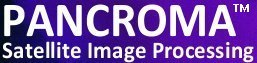See additional satellite image processing articles at www.PANCROMA.com menu selection 'White Papers'

# Using Multiple Band Regression to Improve Pan Sharpened Image Quality

 PANCROMA has utilities for computing multiple linear regression on satellite band files. Such linear regressions can have many uses. One of the most interesting is to conduct image transformations in order to improve the color tones of processed images. The transformation equations used to approximate natural color composites from ASTER and SPOT multispectral composite images are one example. The equation coefficients for these transformations were determined by processing a large number of images and optimizing the coefficients for the general case. As a result, the transformations usually produce acceptable results for most cases but rarely yield ideal results for a particular case. The procedure outlined in this article is designed to create image-specific transformation equations using multiple linear regression to compute the coefficients. The main advantage of this approach is that the transformation equation is optimized for the particular target image of interest, and can often yield improved results over a general transformation equation. The objective of this example will be to improve the color tones of a pan sharpened image produced by the HSI pan sharpening process. However the technique outlined can be used to create a transformation equation for any image, as long as a suitable reference image is available, as will be shown below. As discussed in previous articles and in the PANCROMA Instruction Manual, pan sharpening Landsat multispectral band files using the Landsat panchromatic band can create spectral distortions. This is a result of the characteristics of the Landsat panchromatic sensor. Rather than being sensitive to the visible spectrum, its sensitivity is shifted to longer wavelength electromagnetic radiation, so that it excludes the blue band and includes the near infrared (NIR) band. As a result, pan sharpening with the Landsat panchromatic band yields an image that can show unnatural color tones. One remedy is to use the near infrared band to correct the panchromatic band. The PANCROMA AJISANE and XIONG algorithms do this. Another possible approach is to create a transformation equation that can be used to post-process the image to correct spectral distortions. This is the technique that will be demonstrated in this article. The procedure to create the transformation will be as follows: Create a pan sharpened image using the HSI process Decompose the pan sharpened RGB image into its component blue, green and red band files Resize these band files to match the row and column counts of the original source band files Compute the coefficients of a linear equation that transforms the three resized bands to each of the source band files, individually Use the resulting three equations to transform the pan sharpened component bands to corrected component bands Recombine the transformed bands to create an improved RGB pan sharpened image Important Note: PANCROMA handles zero-value DNs differently, depending on the number of independent variable bands in the regression analysis. For single band and two band regression, i.e. solving Y = a + b1X1 or Y = a + b1X1 + b2X2, the no-data DNs are automatically excluded from the computation. If they were not, the intercept and slope coefficients would be skewed as a result of the preponderance of zero-zero points. For higher level regressions, i.e. for Y = a + b1X1 + b2X2 + b3X3 and for Y = a + b1X1 + b2X2 + b3X3 + b4X4, the no-data DNs are NOT automatically excluded. The reason is that PANCROMA solves the first two sets of equations algebraically and excluding them is relatively easy. PANCROMA computes the higher level regressions using matrix operations and excluding them automatically is a lot messier. To get the best results, you should subset your image sets using PANCROMA subsetting utilities as done in this tutorial to exclude the black collar pixels. The first step is to pan sharpen an image. I used a subset of Landsat L71001086_08620051201_BXX.TIF. I pan sharpened it using the PANCROMA HSI transform by successively opening the three multispectral band files and the panchromatic band, and then selecting 'Pan Sharpening' | 'HSI Transform' | 'Standard Four File' from the Main Menu. The results are shown in the image below.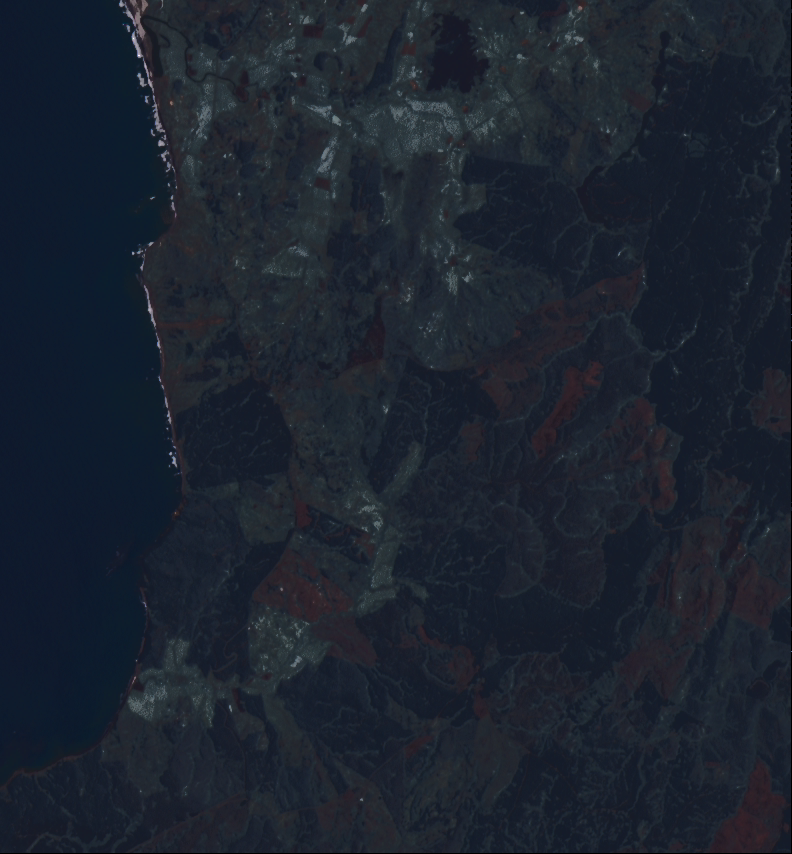The following image is the RGB color composite from the multispectral band files. This is the standard by which color tones will be evaluated. The following image is the RGB color composite from the multispectral band files. This is the standard by which color tones will be evaluated.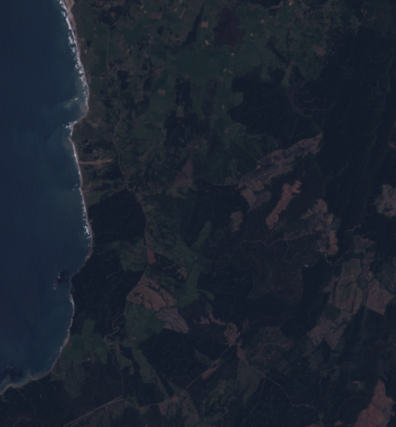The next step is to decompose the pan sharpened RGB image into its component band files. This can be done by opening the RGB image and checking the 'Generate Grayscale Band Images' check box at the bottom of the Main Window. (You can uncheck the 'Generate RGB Color Composite Image' if you want. Then select 'Display One File' | 'Display One Color Composite Image'. When the grayscale band files are created, save them by selecting 'File' | 'Save Grayscale Image' | 'GeoTiff' and supplying a base file name, like 'rawImage'. PANCROMA will automatically apply the correct band number suffix. The next step is to compute the regression coefficients. But before we can do this, the raw images must be resized to match the row and column counts of the source multispectral images. This is necessary because the multiple regression utility wants all input files to match. You can resize the images by opening the three raw pan sharpened images and then selecting 'Pre Process' | 'Resize Images' | 'Resize Three Images'. You will be prompted for the row and column counts. Enter the row and column dimensions of the unprocessed Landsat band files. In this example, the size of the pan sharpened bands was 854 by 792 and the size of the multispectral bands was 427 by 396, i.e. the multispectral row and column counts were half those of the pan sharpened image. After resizing, save as GeoTiffs. My base file name was 'resizedPanSharpenedGrayscale'. Again, PANCROMA will assign the appropriate band number suffixes. Regression coefficients must be computed for each raw band file individually. Then the coefficients will be used to transform the raw band file into a corrected band file. PANCROMA has a utility for regressing three independent variable (bands) against a third dependent variable band, i.e. solving the equation Y = a + b1X1 + b2X2 + b3X3 Where X1, X2 and X3 represent the independent multispectral or hyperspectral band files and Y represents the dependent band file. In order to compute the slope and intercept terms, select 'File' | 'Open' and open the dependent (Y) band first, and then the X1, X2 and X3 bands. Then select 'PreProcess' | 'Multiple Regression' | 'Three Independent Variables'. When the Data Entry box becomes visible, select the sampling interval. (If the sampling interval is one, every DN will be included in the computation and it will take longer. If the interval is 2, every other DN will be computed, etc.) The linear coefficient terms will be reported to the diagnostic screen on the Main Window. They will also automatically be inserted into the Data Entry Form for subsequent use for linear transformations. We will conduct the transformations in three steps as follows. Open the Landsat blue band file. Then open the three resized pan sharpened band files so that four files are opened. Now compute the regression coefficients as described above. I accepted all of the default conditions for this example. After the coefficients are computed, select 'Close Graphics Window and Reset'. Now select 'Pre Process' | 'Band Math' | 'Band Linear Combination' | 'Three Bands'. If you have done everything correctly, you should see the Data Entry form already populated with your regression coefficients, looking something like the form below.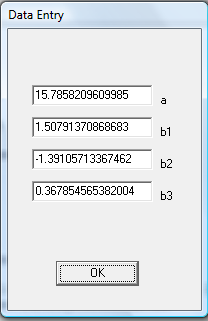Press 'OK'. The transformed grayscale band will be computed and displayed. Save it as a GeoTiff file. I used the base file name 'processedImage1'. Repeat the procedure above for the second Landsat multispectral band (i.e. the green band). That is, open the green band and the three resized raw pan sharpened bands. Compute the next set of coefficients and then transform the raw pan sharpened green band. Finally, repeat the procedure for the red band. What we have done is to create a linear equation that maps the three raw pan sharpened band to the source blue band. In other words, the equation defines how much of the raw blue, green and red bands must be combined in order to make the blue band. Because the coefficients are the result of a regression, the mapping will not be exact for each pixel. But hopefully the mapping will be close enough in order to produce an improved pan sharpened band, and thus an improved pan sharpened image. The only step left is to create an RGB color composite using the processed pan sharpened bands. Select 'Band Combination' | 'Generate RGB True Color Composite'. The result of my efforts is shown below.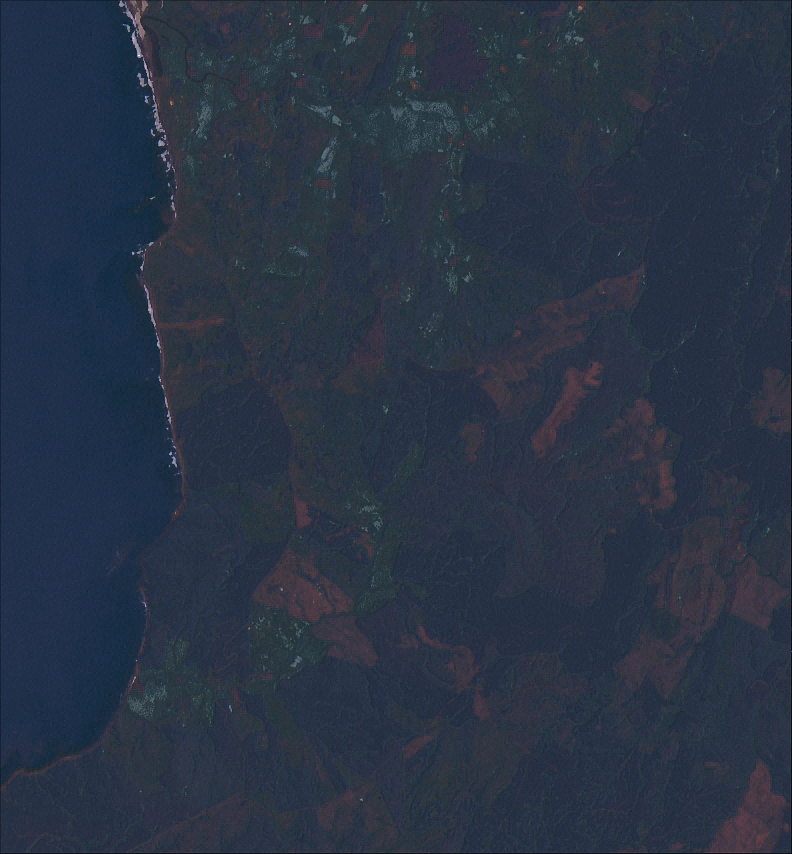Several features of the transformed image are apparent. The color tones are more natural. In addition, the blue highlights caused by the NIR sensitivity of the panchromatic sensor to the high reflectivity of grassland areas is reduced. The processed image is somewhat less sharp than the unprocessed pan sharpened image. This might be improved by using the PANCROMA image processing utilities. I examined the residuals of the regression analysis. Residuals are the difference between the actual band DN and the DN computed by the regression equation. A visual inspection indicated that the fit was not a great one in this case. However it is close enough to illustrate the technique and may even be close enough to be useful. This article has demonstrated the possible advantages of creating image-specific transformation equations in improving RGB image quality. Although this demonstration was for a pan sharpened image, the technique has general application wherever a lower resolution reference image is available for creating transformation coefficients. As mentioned, an issue with this data is that the elevation files are saved mirror flipped around the center latitude line. PANCROMA has a utility for correcting this problem. The elevation data is saved by NASA in GeoTiff format using IEEE floating point 32 bit representation for the elevation postings. It is necessary to flip the elevation postings without changing the georeferencing in order to use the data. To produce a corrected file, select 'File' | 'Open' and open the elevation file. Then select 'DisplayOne File' | 'Display GeoTiff IEEE FP 32 Elevation Image' | 'Flipped'. A rendering of the processed file will appear. You can save the file by selecting 'File' | 'Save Grayscale Image' | 'GeoTiff'. The file will be saved as a GeoTiff DEM (not a raster image).